Learn data science and machine learning by building real-world projects on Jovian

## Analysis of COVID-19 data in Algeria and Comparison: Algeria & Arab countries

### Introduction

The COVID-19 pandemic in Algeria is part of the worldwide pandemic of coronavirus disease 2019 (COVID-19) caused by severe acute respiratory syndrome coronavirus 2 (SARS-CoV-2). The virus was confirmed to have spread to Algeria in February 2020.

The project on Analysis of COVID-19 data in Algeria and Comparison; confirmed cases and deaths, daily and monthly statistics from January to September 2020, analyzed in Algeria, its provinces, and then compared with Arab countries. As follows:

• Data Preparation and Cleaning
• Exploratory Analysis and Visualization
• Part (I): Algeria & its Provinces
• Part (II): Algeria & Arab countries
• Inferences and Conclusion
• References and Future Work
• Call

In this project, I will try to use most of what I have learned in this great course Data Analysis with Python: Zero to Pandas to the analysis of COVID-19 data in my nice country Algeria and Comparised with Arab countries. To come out with results that may benefit Algeria and the world in the future.

## Project Title

In :
``project_name = "Analysis of COVID-19 data in Algeria and Comparison: Algeria & Arab countries" ``
In :
``!pip install jovian --upgrade -q``
In :
``import jovian``
In :
``jovian.commit(project=project_name)``
```[jovian] Attempting to save notebook.. [jovian] Updating notebook "math-nights/analysis-of-covid-19-data-in-algeria-and-comparison-algeria-arab-countries" on https://jovian.ml/ [jovian] Uploading notebook.. [jovian] Capturing environment.. [jovian] Committed successfully! https://jovian.ml/math-nights/analysis-of-covid-19-data-in-algeria-and-comparison-algeria-arab-countries ```
Out:
``'https://jovian.ml/math-nights/analysis-of-covid-19-data-in-algeria-and-comparison-algeria-arab-countries'``

## Data Preparation and Cleaning

#### Importing libraries

• pandas
• matplotlib
• seaborn
• numpy
• jovian
In :
``````import pandas as pd
import matplotlib
import matplotlib.pyplot as plt
import seaborn as sns
%matplotlib inline
import numpy as np``````

#### Configuring styles

In :
``````sns.set_style("darkgrid")
matplotlib.rcParams['font.size'] = 14
matplotlib.rcParams['figure.figsize'] = (9, 5)
matplotlib.rcParams['figure.facecolor'] = '#00000000'``````

#### Algeria location in Africa; map

In :
``````from PIL import Image
img = Image.open('algeria_north_africa.jpg')
plt.grid(False)
plt.title('Algeria')
plt.axis('off')
plt.imshow(img);
# Source: https://www.mapsland.com/africa/algeria/detailed-location-map-of-algeria``````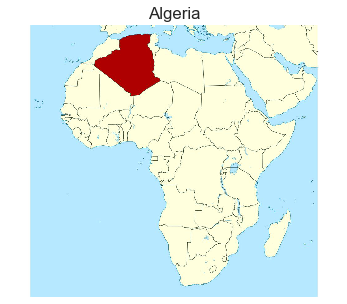#### Reading a file about daily Covid-19 data in Algeria using Pandas

In :
``````covid_dz = pd.read_csv('algeria-covid-data.csv')
covid_dz
``````
Out:

By looking at the data frame:

• The file provides three daywise counts for Covid-19 in Algeria.
• There are: date, new_cases, new_deaths.
• Data is provided for 275 days: from Dec 31, 2019 to Sep 30, 2020.

#### View some basic information about the data frame

In :
``covid_dz.info()``
```<class 'pandas.core.frame.DataFrame'> RangeIndex: 275 entries, 0 to 274 Data columns (total 3 columns): date 275 non-null object new_cases 270 non-null float64 new_deaths 270 non-null float64 dtypes: float64(2), object(1) memory usage: 5.4+ KB ```

It appears that each column contains values of a specific data type.

#### View some statistical information

In :
``covid_dz.describe()``
Out:

In new deaths data, we can see that the mean value is 6, standard deviation 5, minimum 0 & the maximum value is 42.

#### List of columns

In :
``covid_dz.columns.tolist()``
Out:
``['date', 'new_cases', 'new_deaths']``

#### Number of days (dates) in the data frame

In :
``````covid_dz.shape
print('There are {} days in the dataset'.format(covid_dz.shape))``````
```There are 275 days in the dataset ```

#### A random sample of rows from the data frame

In :
``covid_dz.sample(10)``
Out:

#### List of dates

In :
``covid_dz['date']``
Out:
``````0      31/12/2019
1      01/01/2020
2      02/01/2020
3      03/01/2020
4      04/01/2020
...
270    26/09/2020
271    27/09/2020
272    28/09/2020
273    29/09/2020
274    30/09/2020
Name: date, Length: 275, dtype: object``````

There are 275 days: from Dec 31, 2019 to Sep 30, 2020

#### List of New cases

In :
``covid_dz.new_cases``
Out:
``````0        0.0
1        0.0
2        0.0
3        0.0
4        0.0
...
270    175.0
271    160.0
272    153.0
273    146.0
274    155.0
Name: new_cases, Length: 275, dtype: float64``````

#### List of New deaths

In :
``covid_dz.new_deaths``
Out:
``````0      0.0
1      0.0
2      0.0
3      0.0
4      0.0
...
270    4.0
271    4.0
272    3.0
273    5.0
274    7.0
Name: new_deaths, Length: 275, dtype: float64``````

#### Number of missing new cases

In :
``````new_cases_missing = covid_dz.new_cases.isna().sum()
print('There are {} missing new cases in the dataset'.format(new_cases_missing))``````
```There are 5 missing new cases in the dataset ```

#### Number of missing new deaths

In :
``````new_deaths_missing = covid_dz.new_deaths.isna().sum()
print('There are {} missing new deaths in the dataset'.format(new_deaths_missing))``````
```There are 5 missing new deaths in the dataset ```

#### Compare the new cases vs. new deaths

In :
``````compare_dz = covid_dz[['new_cases','new_deaths']]
compare_dz
``````
Out:

#### Date of rise first case

In :
``````covid_first_case = covid_dz.loc[55:60]
covid_first_case
``````
Out:

The first case was on Feb 26, 2020

#### Date of first death

In :
``````covid_first_death = covid_dz.loc[65:75]
covid_first_death
``````
Out:

The first death was on March 13, 2020

#### Dates with the highest new cases

In :
``````highest_cases = covid_dz.sort_values('new_cases', ascending=False).head(10)
highest_cases
``````
Out:
• All dates with the highest new cases are in July
• July 25, 2020 was the day with the highest new cases at all

#### Dates with the highest new deaths

In :
``````highest_deaths = covid_dz.sort_values('new_deaths', ascending=False).head(10)
highest_deaths
``````
Out:
• All dates with the highest new deaths are in April
• April 04, 2020 was the day with the highest new deaths at all

#### Dates before & after April 04, 2020

In :
``covid_dz.loc[90:100]``
Out:

The number of deaths was low, then suddenly increased to 42, and then gradually decreased

#### Dates with the lowest new cases

In :
``````lowest_cases = covid_dz[covid_dz.new_cases > 0].sort_values('new_cases').head(10)
lowest_cases
``````
Out:
• Most dates with the lowest new cases are in March
• Feb 26, March 14, 2020 were the days with the lowest new cases at all

#### Dates with the lowest new deaths

In :
``````lowest_deaths = covid_dz[covid_dz.new_deaths > 0].sort_values('new_deaths').head(10)
lowest_deaths
``````
Out:
• Most dates with the lowest new deaths are in March
• March 13, 14, 18, 19, 20, 28, 2020 were the days with the lowest new deaths at all
In [ ]:
`` ``
In :
``import jovian``
In :
``jovian.commit()``
```[jovian] Attempting to save notebook.. [jovian] Updating notebook "math-nights/analysis-of-covid-19-data-in-algeria-and-comparison-algeria-arab-countries" on https://jovian.ml/ [jovian] Uploading notebook.. [jovian] Capturing environment.. [jovian] Committed successfully! https://jovian.ml/math-nights/analysis-of-covid-19-data-in-algeria-and-comparison-algeria-arab-countries ```
Out:
``'https://jovian.ml/math-nights/analysis-of-covid-19-data-in-algeria-and-comparison-algeria-arab-countries'``

## Exploratory Analysis and Visualization

#### The number of total cases & total deaths in Algeria

In :
``````total_cases = covid_dz.new_cases.sum()
total_deaths = covid_dz.new_deaths.sum()
print('The number of total cases is {} and the number of total deaths is {} in Algeria; Until September 30, 2020 '.format(int(total_cases), int(total_deaths)))``````
```The number of total cases is 51368 and the number of total deaths is 1726 in Algeria; Until September 30, 2020 ```

Pie chart of the number of total cases & total deaths in Algeria

In :
``````labels = 'total cases', 'total deaths'
total = [51368, 1726]
explode = (0, 0)

fig1, ax1 = plt.subplots()
ax1.pie(total, explode=explode, labels=labels, autopct='%1.1f%%', shadow=True, startangle=90)
ax1.axis('equal')
ax1.set_title("COVID-19 Total Cases & Total Deaths in Algeria\n")

plt.show()
``````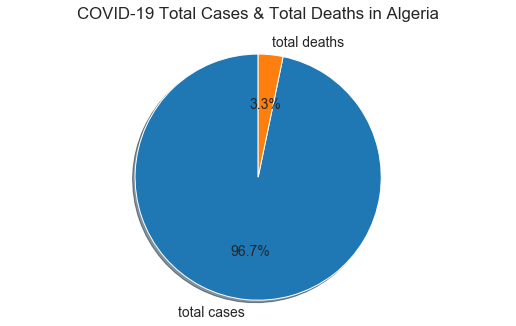#### The average of total cases & total deaths in Algeria

In :
``````avrg_cases = covid_dz.new_cases.mean()
avrg_deaths = covid_dz.new_deaths.mean()
print('The average of total cases is {} and the average of total deaths is {} in Algeria.'.format(int(avrg_cases), int(avrg_deaths)))``````
```The average of total cases is 190 and the average of total deaths is 6 in Algeria. ```

#### The overall death rate in Algeria

In :
``````death_rate = covid_dz.new_deaths.sum() / covid_dz.new_cases.sum()
print("The overall death rate in Algeria is {:.2f} %.".format(death_rate*100))``````
```The overall death rate in Algeria is 3.36 %. ```

#### List of high new cases

In :
``````high_new_cases = covid_dz.new_cases > 600
covid_dz[high_new_cases]
``````
Out:

The highest new cases are greater than 600

#### List of high new deaths

In :
``````high_new_deaths = covid_dz.new_deaths > 20
covid_dz[high_new_deaths]
``````
Out:

The highest new deaths are between 20 and 42

#### Extract dates into separate columns: year, month, day & weekday

In :
``````covid_dz['year'] = pd.DatetimeIndex(covid_dz.date).year
covid_dz['month'] = pd.DatetimeIndex(covid_dz.date).month
covid_dz['day'] = pd.DatetimeIndex(covid_dz.date).day
covid_dz['weekday'] = pd.DatetimeIndex(covid_dz.date).weekday # weekday: the day of the week with Monday=0, Sunday=6
covid_dz
``````
Out:

#### The month with the highest number of new deaths: total cases & total deaths

In :
``````print('July')
covid_dz[covid_dz.month == 7][['new_cases', 'new_deaths']].sum()``````
```July ```
Out:
``````new_cases     12823.0
new_deaths      257.0
dtype: float64``````

#### Summarize the daywise data and create a new data frame with month-wise data

In :
``````covid_month_dz = covid_dz.groupby('month')[['new_cases', 'new_deaths']].sum()
covid_month_dz
``````
Out:

#### The monthly averages

In :
``````covid_month_avrg_dz = covid_dz.groupby('month')[['new_cases', 'new_deaths']].mean()
covid_month_avrg_dz
``````
Out:

#### Calculate the cumulative sum of cases & deaths. Let's add 2 new columns: `total_cases`, `total_deaths`

In :
``````covid_dz['total_cases'] = covid_dz.new_cases.cumsum()
covid_dz['total_deaths'] = covid_dz.new_deaths.cumsum()
covid_dz
``````
Out:

#### Plot the new cases per day

In :
``````plt.figure(figsize=(12,6))
plt.title('COVID-19 Daily New Cases in Algeria')
covid_dz.new_cases.plot(color='purple');``````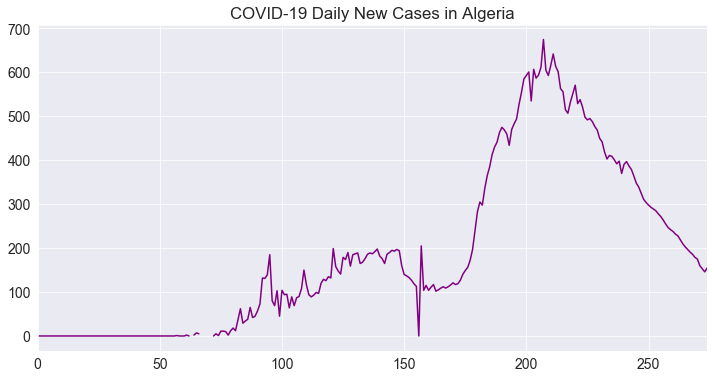In :
``````plt.figure(figsize=(12,6))
plt.title('COVID-19 Daily New Cases in Algeria')
covid_dz.new_cases.plot(kind='area', color='purple');``````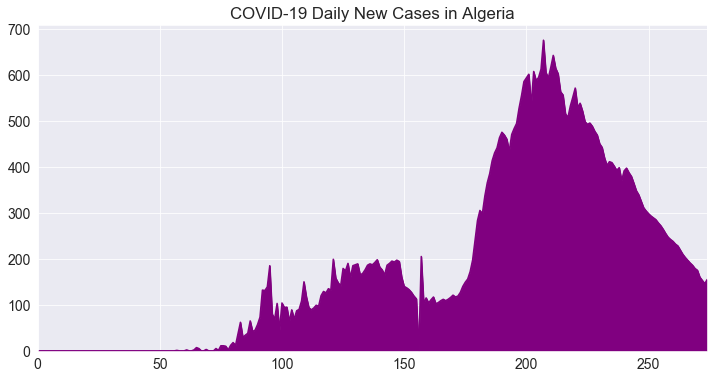• The number of cases was low, then gradually increased, then decreased slightly, then suddenly increased to reach it's maximum, then gradually decreased.
• We notice that the disease has two waves of spread:
• The First: the speed of spread was medium
• The second: the speed of spread was very fast
In :
``````plt.figure(figsize=(12,6))
plt.title('New Cases Range in Algeria')
plt.xlabel('New Cases')
plt.ylabel('Values')
plt.hist(covid_dz.new_cases, bins=np.arange(1, 675, 10), color='orchid');``````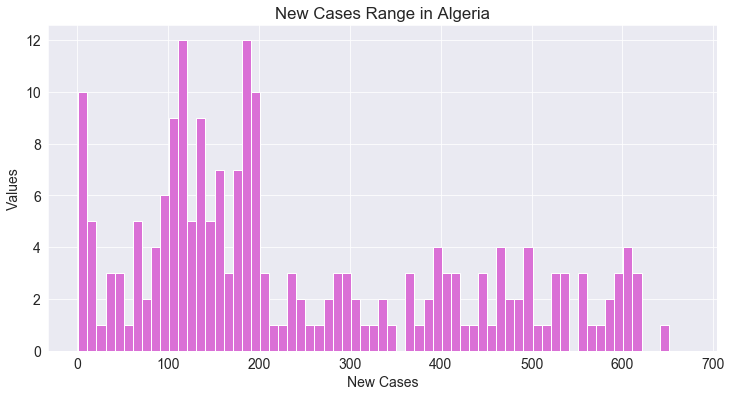Most new cases are lying in the range of fewer than 200 cases per day

#### Plot the new deaths per day

In :
``````plt.figure(figsize=(12,6))
plt.title('COVID-19 Daily New Deaths in Algeria')
covid_dz.new_deaths.plot(color='red');``````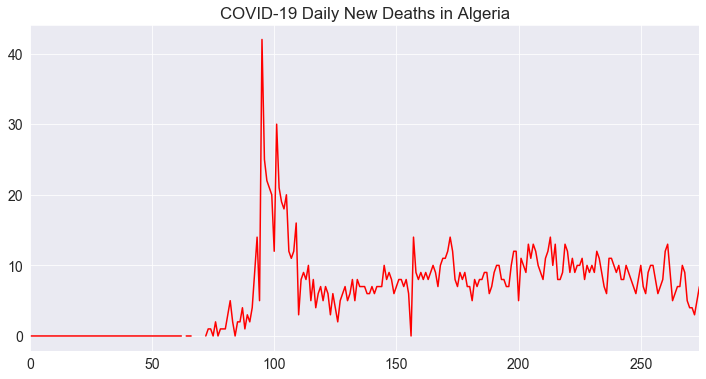In :
``````plt.figure(figsize=(12,6))
plt.title('COVID-19 Daily New Deaths in Algeria')
covid_dz.new_deaths.plot(kind='area', color='red');``````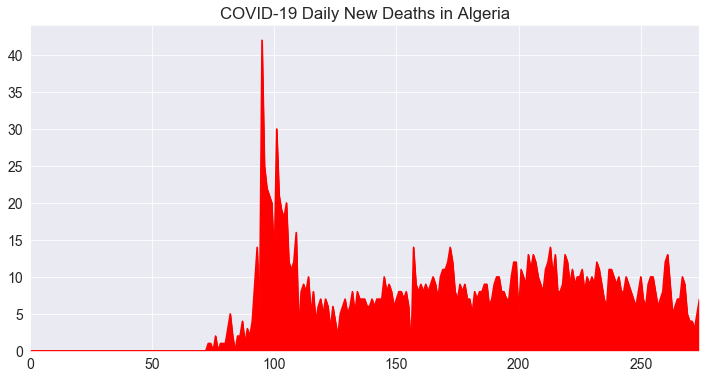The number of deaths was low, then it suddenly increased to its maximum, then began to gradually decrease, then began to fluctuate within a range of less than 15

In :
``````plt.figure(figsize=(12,6))
plt.title('New Deaths Range in Algeria')
plt.xlabel('New Deaths')
plt.ylabel('Values')
plt.hist(covid_dz.new_deaths, bins=np.arange(1, 42, 1), color='hotpink');``````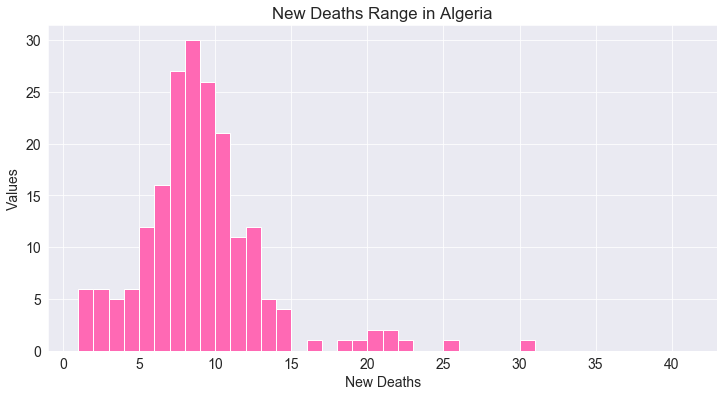Most new deaths are lying in the range of fewer than 15 deaths per day

#### Compare the new cases vs. new deaths

In :
``````plt.figure(figsize=(12,6))
plt.title('COVID-19 Daily New Cases & Deaths in Algeria')
covid_dz.new_cases.plot(color='purple')
covid_dz.new_deaths.plot(color='red');``````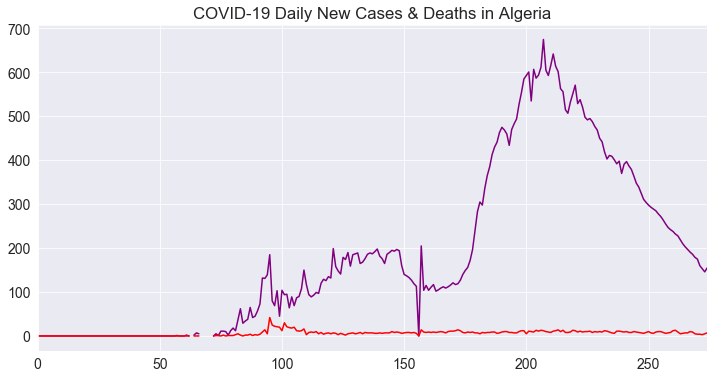In :
``````plt.figure(figsize=(12,6))
plt.title('COVID-19 Daily New Cases & Deaths in Algeria')
covid_dz.new_cases.plot(kind='area', color='purple')
covid_dz.new_deaths.plot(kind='area', color='red');``````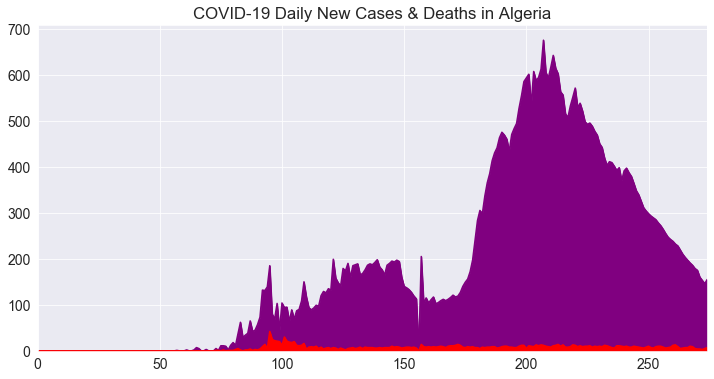The number of deaths is very small compared to the very large number of cases

#### compare the total cases vs. total deaths

In :
``````plt.figure(figsize=(12,6))
plt.title('COVID-19 Total cumulatively: Daily New Cases & New Deaths in Algeria')
covid_dz.total_cases.plot(color='purple')
covid_dz.total_deaths.plot(color='red');``````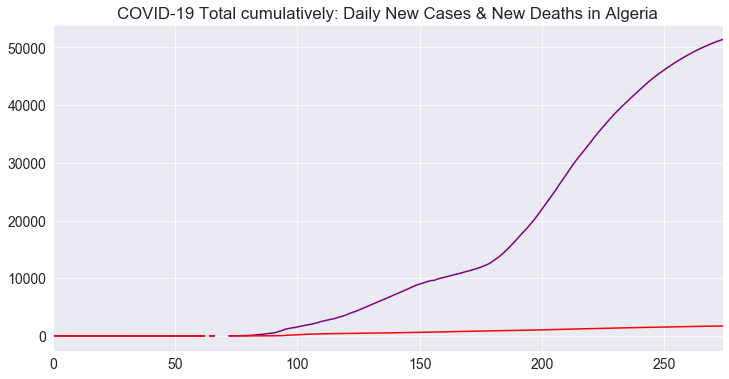The number of deaths is very small, over all days, which is a good sign.

In :
``````plt.figure(figsize=(12,6))
plt.title('COVID-19 Total cumulatively: Daily New Cases & New Deaths in Algeria')
covid_dz.total_cases.plot(kind='area', color='purple')
covid_dz.total_deaths.plot(kind='area',color='red');``````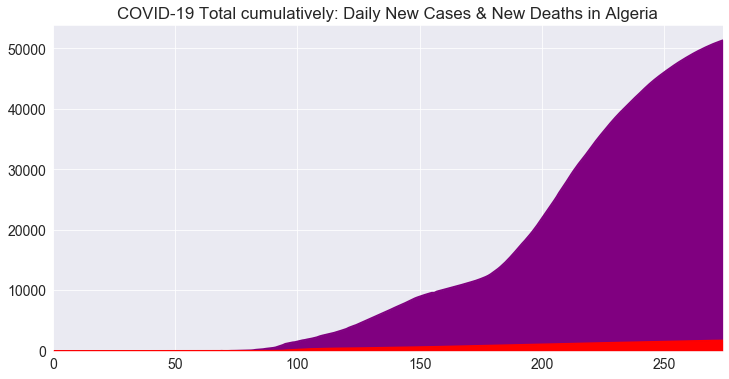That confirms that most of those who were infected have recovered

#### Plot the new cases per month

In :
``````plt.figure(figsize=(12,6))
plt.title('COVID-19 Monthly New Cases in Algeria')
covid_month_dz.new_cases.plot(color='blueviolet');``````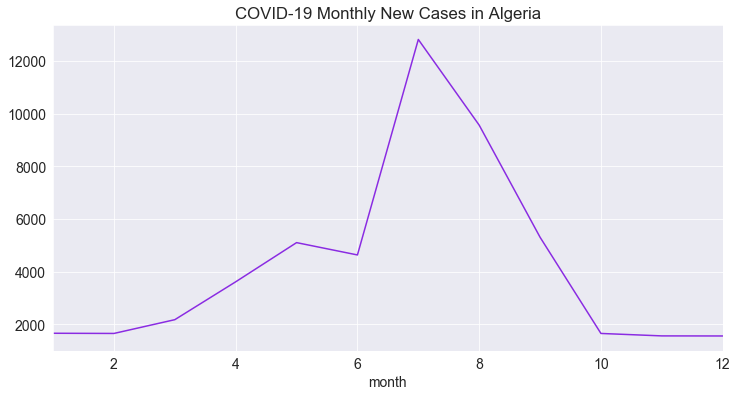In :
``````plt.figure(figsize=(12,6))
plt.title('COVID-19 Monthly New Cases in Algeria')
covid_month_dz.new_cases.plot(kind='area', color='blueviolet');``````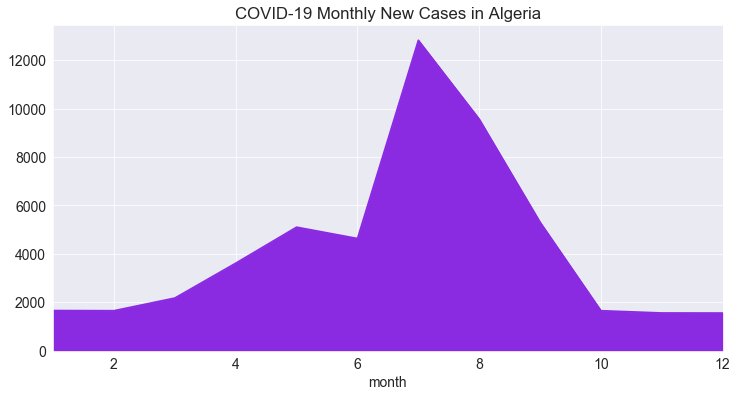In :
``````plt.figure(figsize=(12,6))
plt.title('COVID-19 Monthly New Cases in Algeria')
covid_month_dz.new_cases.plot(kind='barh', color='blueviolet' );``````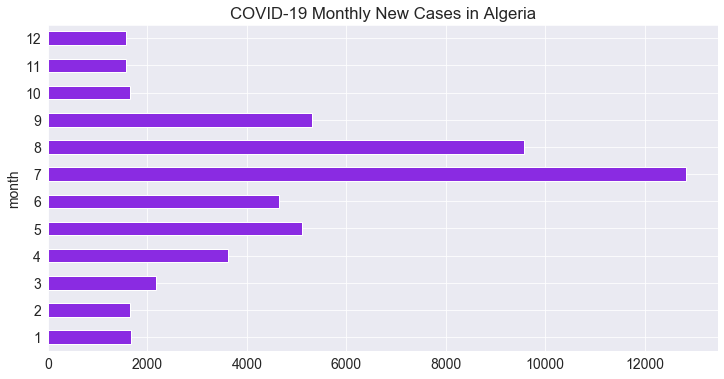• The most cases are in months: July & August
• The most cases are in the summer season
• That is, Covid-19 spreads in the summer when the temperature rates are high, as well

#### Plot the new deaths per month

In :
``````plt.figure(figsize=(12,6))
plt.title('COVID-19 Monthly New Deaths in Algeria')
covid_month_dz.new_deaths.plot(color='orangered');``````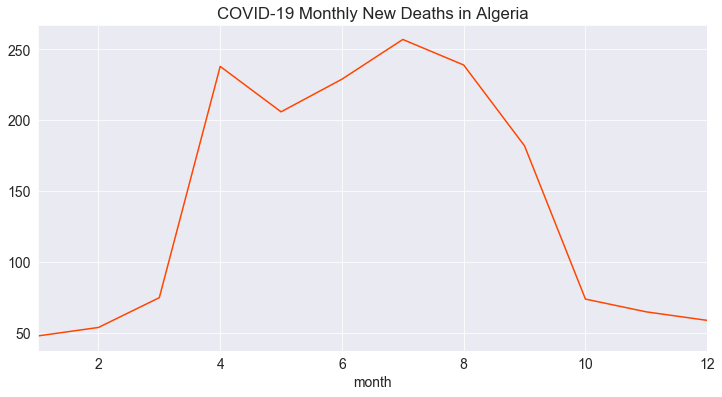In :
``````plt.figure(figsize=(12,6))
plt.title('COVID-19 Monthly New Deaths in Algeria')
covid_month_dz.new_deaths.plot(kind='area', color='orangered');``````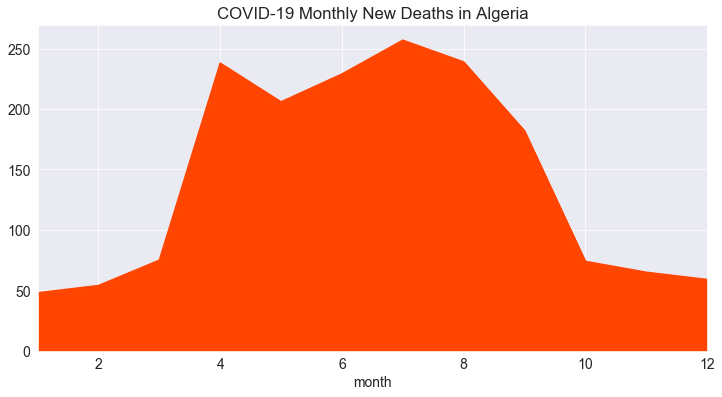In :
``````plt.figure(figsize=(12,6))
plt.title('COVID-19 Monthly New Deaths in Algeria')
covid_month_dz.new_deaths.plot(kind='barh', color='orangered');``````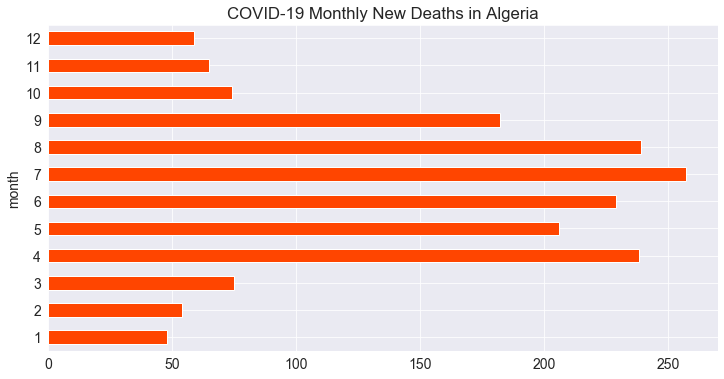• The most deaths are between April & September
• The most deaths are in the summer season
• That is, Covid-19 spreads in the summer as well

#### Compare per month: the new cases vs. new deaths

In :
``````plt.figure(figsize=(12,6))
plt.title('COVID-19 Monthly New Cases & New Deaths in Algeria')
covid_month_dz.new_cases.plot(color='blueviolet')
covid_month_dz.new_deaths.plot(color='orangered');``````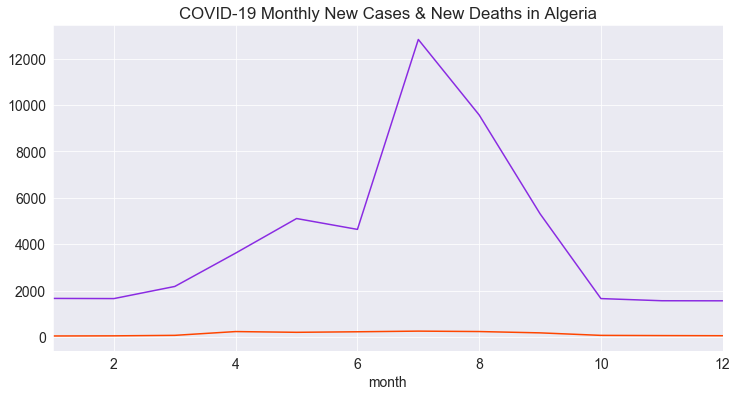The number of deaths is very small, over all months, which is a good sign.

In :
``````plt.figure(figsize=(12,6))
plt.title('COVID-19 Monthly New Cases & New Deaths in Algeria')
covid_month_dz.new_cases.plot(kind='area', color='blueviolet')
covid_month_dz.new_deaths.plot(kind='area', color='orangered');``````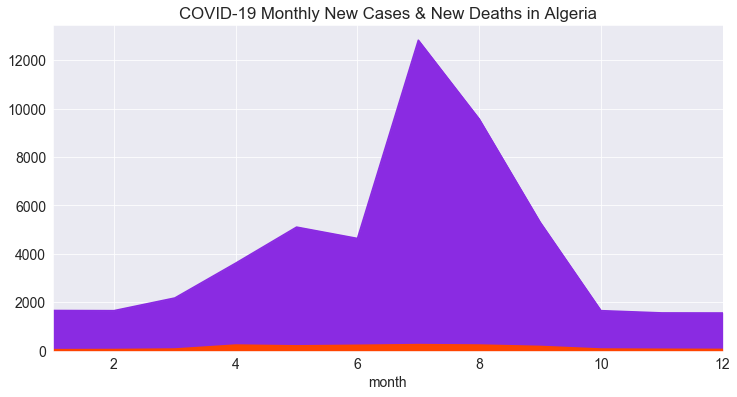That confirms that most of those who were infected have recovered

#### Python list showing months, new cases & new deaths

In :
``````months = ['Jan', 'Feb', 'Mar', 'Apr', 'May', 'Jun', 'Jul', 'Aug', 'Sep']
new_cases = [1666, 1659, 2182, 3620, 5108, 4640, 12823, 9569, 5313]
new_deaths = [48, 54, 75, 238, 206, 229, 257, 239, 182]``````

#### Line Chart: Monthly New Cases in Algeria

In :
``````plt.figure(figsize=(12,6))
plt.plot(months, new_cases,'s--b')

plt.xlabel('Months')
plt.ylabel('New Cases')

plt.title("COVID-19 Monthly New Cases in Algeria")
plt.legend(['new cases']);``````